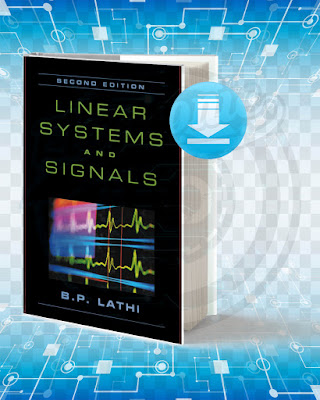## Linear Systems and Signals.

### Picture Of The Book:Linear Systems and Signals

Incorporating new problems and examples, the second edition of "Linear Systems" features MATLAB material in each chapter and at the back of the book. It gives clear descriptions of linear systems and uses mathematics not only to prove the axiomatic theory, but also to enhance physical and intuitive understanding.

Title: Linear Systems and Signals.
Language: English.
Size: 26.4 Mb.
Pages: 689.
Format: Pdf.
Year: 2005.
Edition: 2.
Author: B. P. Lathi.

### Contents Of The Book:

Chapter 1: Introduction.
Chapters 2 and 3: Time-domain analysis of linear time-invariant (LTI) systems.
Chapters 4 and 5: Frequency-domain (transform) analysis of LTI systems.
Chapters 6, 7, 8, and 9: Signal analysis.
Chapter 10: State-space analysis of LTI systems.15 sec